# Ricci curvature

(diff) ← Older revision | Latest revision (diff) | Newer revision → (diff)

of a Riemannian manifoldat a point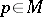A number corresponding to each one-dimensional subspace of the tangent spaceby the formula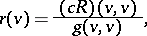whereis the Ricci tensor,is a vector generating the one-dimensional subspace andis the metric tensor of the Riemannian manifold. The Ricci curvature can be expressed in terms of the sectional curvatures of. Let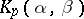be the sectional curvature at the pointin the direction of the surface element defined by the vectorsand, let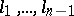be normalized vectors orthogonal to each other and to the vector, and letbe the dimension of; thenFor manifoldsof dimension greater than two the following proposition is valid: If the Ricci curvature at a pointhas one and the same valuein all directions, then the Ricci curvature has one and the same valueat all points of the manifold. Manifolds of constant Ricci curvature are called Einstein spaces. The Ricci tensor of an Einstein space is of the form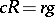, whereis the Ricci curvature. For an Einstein space the following equality holds:where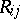,are the covariant and contravariant components of the Ricci tensor,is the dimension of the space andis the scalar curvature of the space.

The Ricci curvature can be defined by similar formulas also on pseudo-Riemannian manifolds; in this case the vector is assumed to be anisotropic.

From the Ricci curvature the Ricci tensor can be recovered uniquely: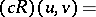How to Cite This Entry:
Ricci curvature. Encyclopedia of Mathematics. URL: http://encyclopediaofmath.org/index.php?title=Ricci_curvature&oldid=12970
This article was adapted from an original article by L.A. Sidorov (originator), which appeared in Encyclopedia of Mathematics - ISBN 1402006098. See original article